[Next]: Projects interconnecting research groups
 [Up]: Project descriptions
 [Previous]: Stress analysis of thin wafers
[Contents]   [Index]

## Kinetic solution of sample initial and boundary value problems

Collaborator: W. Dreyer , M. Herrmann , Sh. Qamar

Cooperation with: M. Kunik (Otto-von-Guericke-Universität Magdeburg), M. Junk (Universität Kaiserslautern)

Supported by: DFG: Kinetische Behandlung von ausgewählten Anfangs- und Randwertproblemen'' (Kinetic solution of sample initial and boundary value problems), Priority Program Analysis und Numerik von Erhaltungsgleichungen'' (ANumE -- Analysis and numerics for conservation laws)

Description: At low temperatures the evolution of heat in crystalline solids is carried by phonons. In particular, the Fourier theory of heat fails to describe heat conduction at low temperatures. The evolution of a phonon gas is governed by the Boltzmann-Peierls equation which is a kinetic equation for the phase density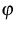of a phonon gas. For more details we refer to  and . The Boltzmann-Peierls equation may be written as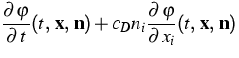=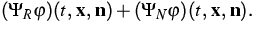(1)
Here t,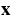and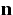denote the time, the space and the normal wave vector, respectively. The collision operators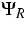and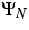are relaxation operators and model the phonon-phonon interactions as well as the influence of the lattice.

The Boltzmann-Peierls equation implies a hierarchy of balance equations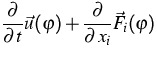=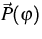(2)
for densities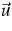, fluxes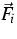and productions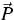.The extended thermodynamics uses the kinetic entropy h, which here reads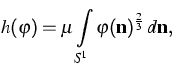(3)
and the Maximum Entropy Principle (MEP) in order to close a finite system of balance equations. There result symmetric hyperbolic systems of PDEs.

The main problems we investigate in this project may be summarized as follows
1.
Existence of MEP operators and properties of the corresponding moment systems;
2.
Kinetic approximations for the MEP moment systems that have the form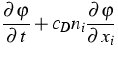=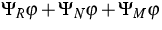(4)

with a suitable relaxation operator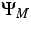;
3.
Kinetic schemes for the kinetic equations as well as for the moment systems;
4.
Mathematical justification of the thermodynamical strategy to replace a kinetic equation by a hyperbolic moment system.
In the last year we concentrated on the mathematical properties of the above-mentioned nonlinear operators and equations. We obtained partial results concerning
1.
Estimates that control Lp bounds for MEP phase densities;
2.
Local existence and global uniqueness results for the Boltzmann-Peierls equation and for the kinetic approximations of MEP moment systems;
3.
Modified collision and MEP operators leading to global existence results.

References:

1. W. DREYER, M. HERRMANN, M. KUNIK, Kinetic schemes and initial boundary value problems for the Euler system, Transport Theory Statist. Phys., 31 (2002), pp. 1-33.
2., Kinetic solutions of the Boltzmann-Peierls equation and its moment systems,
WIAS Preprint no. 709, 2001.
3. W. DREYER, M. JUNK, M. KUNIK, On the approximation of kinetic equations by moment systems, Nonlinearity, 14 (2001), pp. 881-906.
4. W. DREYER, M. KUNIK, Initial and boundary value problems of hyperbolic heat conduction, Contin. Mech. Thermodyn. 11 (1999), pp. 227-245.
5. W. DREYER, M. KUNIK, Kinetische Behandlung von ausgewählten hyperbolischen Anfangs- und Randwertproblemen, DFG Proposal DR 401/2-2.
6. W. DREYER, H. STRUCHTRUP, Heat pulse experiments revisited, Contin. Mech. Thermodyn., 5 (1993), pp. 3-50.
7. M. KUNIK, S. QAMAR, G. WARNECKE, Kinetic schemes for the relativistic gas dynamics, Preprint no. 40, Otto-von-Guericke University, Magdeburg, 2002.

 [Next]: Projects interconnecting research groups
 [Up]: Project descriptions
 [Previous]: Stress analysis of thin wafers
[Contents]   [Index]

LaTeX typesetting by I. Bremer
5/16/2003